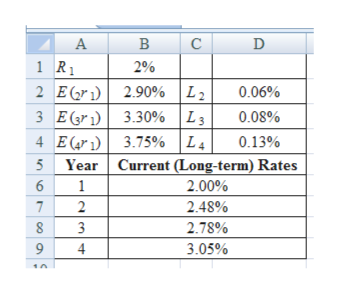Based on economists’ forecasts and analysis, 1-year Treasury bill rates and liquidity premiums for the next four years are expected to be as follows:  R1  =2.00%      E(2r1)=2.90% L2=0.06%  E(3r1)=3.30% L3=0.08%  E(4r1)=3.75% L4=0.13%          Using the liquidity premium theory, determine the current (long-term) rates. (Do not round intermediate calculations. Round your answers to 2 decimal places.)Based on economists’ forecasts and analysis, 1-year Treasury bill rates and liquidity premiums for the next four years are expected to be as follows: R1  =2.00%      E(2r1)=2.90% L2=0.06%  E(3r1)=3.30% L3=0.08%  E(4r1)=3.75% L4=0.13%          Using the liquidity premium theory, determine the current (long-term) rates. (Do not round intermediate calculations. Round your answers to 2 decimal places.)YearsCurrent (Long-term) Rates1 %2 %3 %4 %

Question

Based on economists’ forecasts and analysis, 1-year Treasury bill rates and liquidity premiums for the next four years are expected to be as follows:

 R1 = 2.00 % E(2r1) = 2.90 % L2 = 0.06 % E(3r1) = 3.30 % L3 = 0.08 % E(4r1) = 3.75 % L4 = 0.13 %

Using the liquidity premium theory, determine the current (long-term) rates. (Do not round intermediate calculations. Round your answers to 2 decimal places.)

Based on economists’ forecasts and analysis, 1-year Treasury bill rates and liquidity premiums for the next four years are expected to be as follows:

 R1 = 2.00 % E(2r1) = 2.90 % L2 = 0.06 % E(3r1) = 3.30 % L3 = 0.08 % E(4r1) = 3.75 % L4 = 0.13 %

Using the liquidity premium theory, determine the current (long-term) rates. (Do not round intermediate calculations. Round your answers to 2 decimal places.)

 Years Current (Long-term) Rates 1 % 2 % 3 % 4 %

Step 1

Calculation of Current Long-Term Rates:

The current long-term rates for Year 1 is 2.00%, for Year 2 is 2.48%, for Year 3 is 2.78% and for Year 4 is 3.05%.

...help_outlineImage TranscriptioncloseB C 2% 1R 2 Er2.90% |L2 3 E(gr1) 3.30% L3 4 E(ar 3.75% L4 5Year Current (Long-term) Rates 0.06% 0.08% 0.13% 1 2.00% 7 2 2.48% 2.78% 4 3.05% fullscreen

Want to see the full answer?

See Solution

Want to see this answer and more?

Our solutions are written by experts, many with advanced degrees, and available 24/7

See Solution
Tagged in